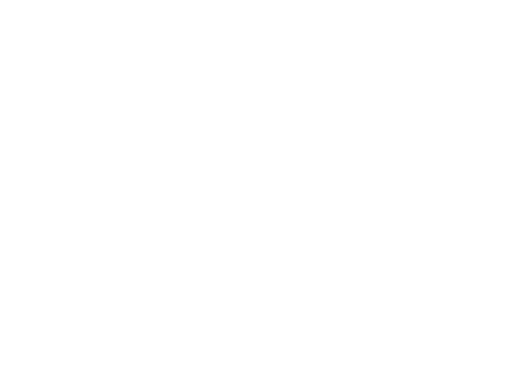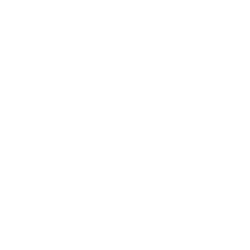# A Crash Course in Fractions on the ACT Math Test

A fraction consists of a numerator, a fraction bar, and a denominator. To add or subtract fractions, you just need to find a common denominator. For example ¼ + 1/3 = 3/12 + 4/12 = 7/12.All fractions are rational numbers. A rational number is a number that can be expressed as a ratio of two integers. Therefore all integers are also rational numbers. Rational numbers either have no decimal (4/1), or have a terminating or repeating decimal (1/4 = .25, 1/3 = .3333).

Equivalent fractions are fractions that simplify to the same form. For example, 6/8, and ¾. In a fraction, when you multiply the numerator and the denominator by the same non-zero integer, it’s like multiplying the entire fraction by 1. The value of the fraction doesn’t change.

To simplify fractions, you need to understand the LCM, lowest common multiple, and the LCD, least common denominator. The LCD is the LCM (the smallest number that is also a multiple of both integers). For example, if we were to solve: 2/3 + ¼  – 1/2. The LCD is going to be the smallest number that 3, 4, and 2 divide into evenly. Since 2 is already a factor of 4, we can choose 12. Since 3, 4, and 2 will all evenly divide into 12.

When you multiply fractions, you can multiply the numerators straight across, then the denominators straight across. Before you multiply, you can also cancel out numbers across the numerators and denominators. For example: 1/5 x 5/6 x 12/2 x 1/3 = ?. One way to start if by crossing out the 5 in the denominator of the first fraction, and the 5 in the numerator of the second fraction. That gives us:

1/1 x 1/6 x 12/2 x 1/3. It’s a much easier question now, and we can cross out the 6 in the denominator of the 2nd fraction if we divide it out of 12, the numerator in the third fraction to give us:

1/1 x 1/1 x 2/2 x 1/3 = 1/3.

A complex fraction is one that contains fractions in the numerator and the denominator. For these, find the LCM of ALL the denominators to simplify.

## Author

•Magoosh is a company of passionate educators, located in Berkeley, CA. We are here to provide you with everything you need to prep for the ACT, SAT, GRE, GMAT, and TOEFL! Be sure to follow us on Twitter @Magoosh.